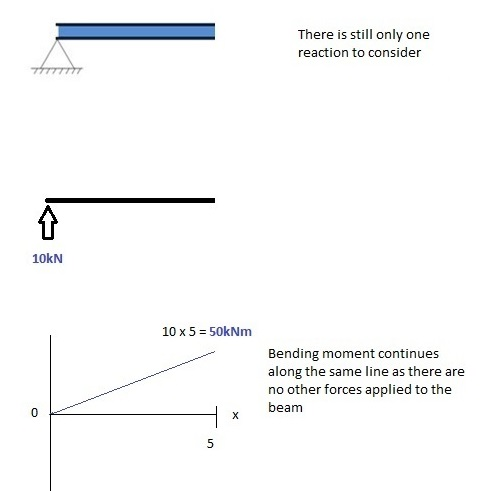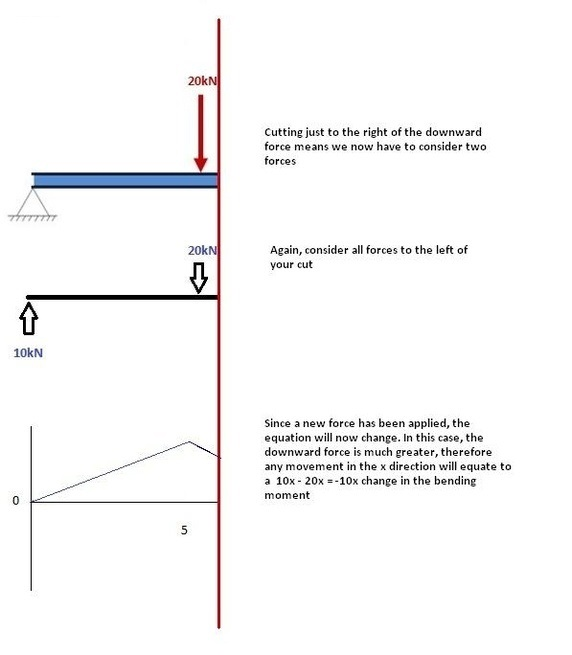The Bending Moment diagram (B.M.D) is a graph showing the variation of bending moment along a beam. The positive Bending Moment (BM) is drawn above the arbitrary reference line while the negative bending moment is drawn below it and a line joining the extremities of the ordinate at salient points from the B.M.D.

The steps to draw Bending Moment diagram (B.M.D) are as follows:
• Calculate reactions at supports and draw Free Body diagram (F.B.D)
Calculate the reactions at the support. After calculating the reactions at support draw the free body diagram (F.B.D) and Shear force diagram underneath the beam.• From left to right, make "cuts" before and after each reaction/load
To calculate the bending moment of a beam, we must work in the same way we did for the shear force diagram. Starting at x = 0, we will move across the beam and calculate the bending moment at each point.

CUT-1:

Make a "cut" just after the first reaction of the beam. In our example:So, when we cut the beam, we only consider the forces that are applied to the left of our cut. In this case we have a 10 KN force in the upward direction. Now as you recall, a bending moment is simply the force X distance. So, as we move further from the force, the magnitude of the bending moment will increase. We can see this in our B.M.D. The equation for this part of our bending moment is:
-M(x) = 10 (-x)
M(x) = 10x

CUT-2:

This cut is made just before the second force along the beam. Since there are no other loads applied between the first and second cut, the bending moment equation will remain the same. This means we can calculate the maximum bending moment (in this case at the midpoint, or x=5) by simply substituting x=5 into the above equation.CUT-3:

This cut is made just after the second force along the beam. Now we have two forces that act to the left of our cut: a 10 KN support reaction and a -20 KN downward acting load. So now we must consider both these forces as we progress along our beam. For every meter we move across the beam, there will a +10 KNm moment added from the first force and -20 KNm from the second. So after the point x=5, our Bending Moment Equation becomes:

M(x) = 50 +10(x-5) - 20(x-5)
M(x) = 50 -10(x-5) for 5 ≤ x ≤ 10

Note: The reason we write (x-5) is because we want to know the distance from the point x=5 only. Anything before this point uses a previous equation.CUT-4:

Again, let's move across to the right of our beam and make a cut just before our next force. In this case, our next cut will occur just before the reaction from right support. Since there are no other forces between the support and our previous cut, the equation will remain the same.

M(x) = 50 -10(x-5) for 5 ≤ x≤ 10

and let's substitute x=10 into this to find the find bending moment at the end of the beam.

M(x) = 50 - 10(10-5) = 0 KNm

This makes perfect sense. Since our beam is static (and not rotation) it makes sense that our beam should have zero moment at this point when we consider all our forces. It also satisfies one of our initial conditions, that the sum of moments at a support is equal to zero.